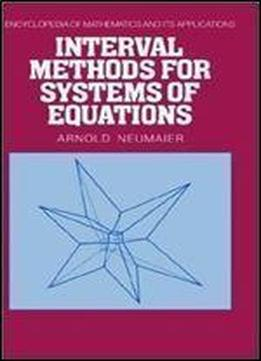# Interval Methods For Systems Of Equations (encyclopedia Of Mathematics And Its Applications, Vol. 37) by Arnold Neumaier / 1991 / English / PDF

An interval is a natural way of specifying a number that is specified only within certain tolerances. Interval analysis consists of the tools and methods needed to solve linear and nonlinear systems of equations in the presence of data uncertainties. Applications include the sensitivity analysis of solutions of equations depending on parameters, the solution of global nonlinear problems, and the verification of results obtained by finite-precision arithmetic.

views: 190